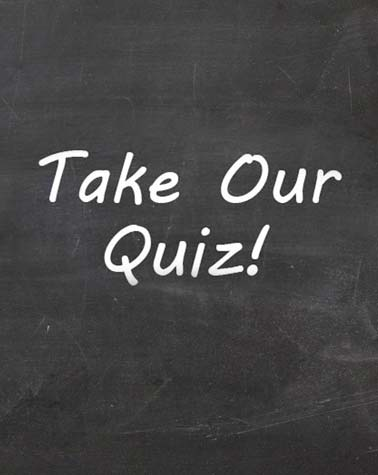# True Or False Chapter 10 - Cause And Effect: Correlation And Regression

11 Questions | Total Attempts: 96Settings• 1.
A graphical picture of bivariate data is called a line diagram.
• A.

True

• B.

False

• 2.
Correlation measures the strength of the association between two variables.
• A.

True

• B.

False

• 3.
Regression defines the relationship between the two variables.
• A.

True

• B.

False

• 4.
Correlation is measured on a scale from 0 to 1.
• A.

True

• B.

False

• 5.
The least squares regression line maximises the sum of the squared errors.
• A.

True

• B.

False

• 6.
A perfect linear relationship between two variables means that all the points lie on a straight line.
• A.

True

• B.

False

• 7.
Spearman's rank correlation coefficient is used for nominal data.
• A.

True

• B.

False

• 8.
A high correlation confirms a causal relationship.
• A.

True

• B.

False

• 9.
A negative correlation coefficient means that there is no association between the two variables.
• A.

True

• B.

False

• 10.
Pearson's product moment correlation coefficient can only be calculated for numerical data.
• A.

True

• B.

False

• 11.
The coefficient b in the linear regression model represents the slope of the regression line.
• A.

True

• B.

False